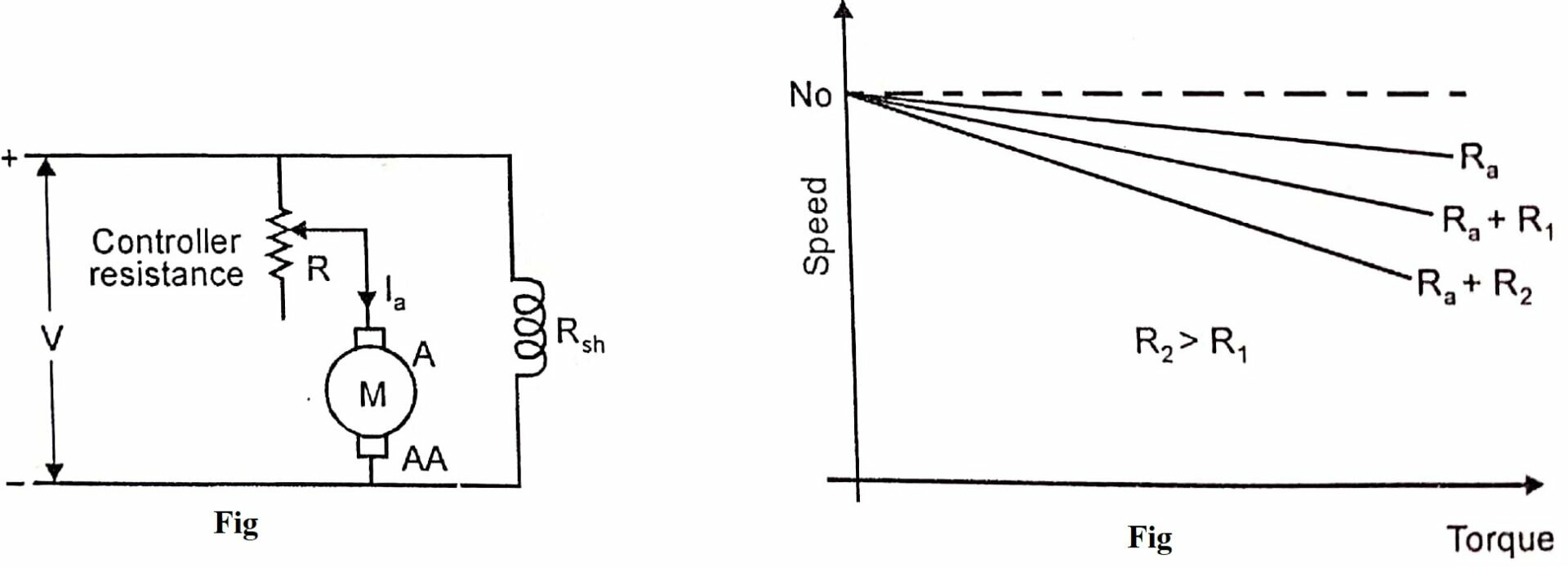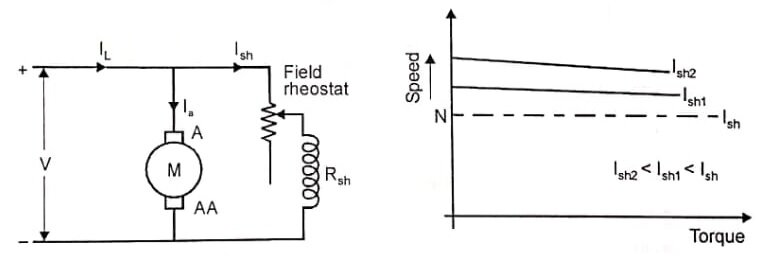# Speed Control of DC Shunt Motor – theteche.com

## Mention speed control methods of DC shunt motors?

1. Armature control method, 2. Field control method, 3. Voltage control method

The speed of a dc motor is given by

$N=\frac{V-I_{a} R_{a}}{Z \phi}\left(\frac{A}{P}\right) \text { (or) } \quad N=\frac{K\left(V-I_{a} R_{a}\right)}{\phi}$

where  V = applied voltage, Ia = armature current
Ra = armature resistance, Φ = flux per pole, K = contant

From the above equation, three methods of speed controls are possible

1. By varying the resistance in the armature circuit (Rheostatic control)
2. By varying the flux (Flux control)
3. By varying the applied voltage (Voltage control)

#### By varying the resistance in the armature circuit

A variable resistance R is connected in series with armature circuit as shown in figure. Here, the input voltage V is constant. The speed of the motor can be controlled by varying the resistor R. So the speed equation becomes

$N=\frac{K\left[V-I_{a}\left(R_{a}+R\right)\right]}{\phi}$

By increasing the controller resistance R, the potential drop across the armature is decreased. Therefore the motor speed also decreases. This method of speed control is applicable only for speed lower than no load speed (base speed). The speed torque characteristic curve of a dc shunt motor with varying value of resistance in armature circuit is shown in figure.From the figure, it is clear that by increasing the armature circuit resistance the speed can be decreased.

Simple method of speed control.

• Here, the input power is not changed (VI). i.e., input is constant. The output power is Eb Ia. It becomes less. Therefore for lower speeds more and more power is wasted in this controller resistance. Hence this method of speed control is highly inefficient.
• Another disadvantage of this method is that the change in speed with the change in load becomes large.

#### By Varying the flux

Another method of speed control of dc shunt motor is flux control method. From the equation (8), the speed is inversely proportional to flux.

N α 1/ΦBy varying the flux, the motor speed can be varied. The flux of a dc motor can be changed by changing the field current (Ish). It is obtained by a variable resistance connected in series with shunt field winding as shown in figure.

By varying the field circuit resistance, the shunt field current can only be decreased. i.e., the flux will be decreased. Thus motor speed can be increased by decreasing the flux. This method of speed control can be used for increasing the speed of the motor above its rated speed (base speed).

Here, the field current Ish is less. Therefore, the shunt field rheostat has to carry only a small current and I²R loss is also less. But this method of speed control cannot be used to obtain large variations of speed. The speed-torque characteristic curve of a dc shunt motor for variation of field current is shown in figure.

#### Ward-Leonard control system

The Ward-Leonard speed control system is shown in figure (a). This system, introduced in the 1890 s, was a significant step in the evolution of dc drives. This system is mainly used where very sensitive speed is required as for electric excavators, elevators, colliery winders and the main drives in steel mills and paper mills.

It consists of three dc machines i.e., two dc motors and one dc generator. The system uses a motor- generator (M1-G) set. The dc drive motor M1 is the main motor, directly coupled to the dc generator G.DC supply is given to the armature terminal as well as shunt field winding.

The M-G set runs at constant speed. The voltage of the generator can be varied from zero to maximum value by means of its field regulator. The generated dc voltage is fed to the controlled dc motor (M2).

The direction of rotation of the controlled dc motor M2 can be changed by reversing the direction of the field current of generator G. It is done by using Reversing Switch (RS) and the generated voltage can be reversed and the motor rotates in reverse direction. This method of speed control is combination of armature control and flux control. The armature voltage control can be achieved by varying the field of dc generator.

The flux control method can be achieved, by varying the field of the controlled dc motor. The Ward Leonard system provides a constant torque as well as constant horse power drive as shown in figure . In the constant torque operating mode, the field flux of the controlled dc motor (drive motor) is kept constant and the armature voltage is controlled. In the constant horse power operating mode, the armature voltage is kept constant and the field current is controlled.

The advantages of this method are

1. Full forward and reverse speed can be achieved.
2. A wide range of speed control is possible.
3. Short-time overload capacity is large.

The disadvantages of the Ward-Leonard drive are

1. High initial cost.
2. The overall efficiency is low, less than  because of an additional M.G. set.
3. Costly foundation and a large amount of space is required.
4. The drive produces noise.
5. It requires frequent maintenance.